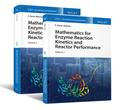John Wiley & Sons Mathematics for Enzyme Reaction Kinetics and Reactor Performance, 2 Volume SetMathematics for Enzyme Reaction Kinetics and Reactor Performance is the first set in a unique 11 vol.. Product #: 978-1-119-49028-9 Regular price: \$285.05 \$285.05 In Stock

# Mathematics for Enzyme Reaction Kinetics and Reactor Performance, 2 Volume Set

Enzyme Reaction Engineering1. Edition April 2020
1072 Pages, Hardcover
Wiley & Sons Ltd

ISBN: 978-1-119-49028-9
John Wiley & Sons

### Price: 305,00 €

estimated price

Price incl. VAT, excl. Shipping

Mathematics for Enzyme Reaction Kinetics and Reactor Performance is the first set in a unique 11 volume-collection on Enzyme Reactor Engineering. This two volume-set relates specifically to the wide mathematical background required for systematic and rational simulation of both reaction kinetics and reactor performance; and to fully understand and capitalize on the modelling concepts developed. It accordingly reviews basic and useful concepts of Algebra (first volume), and Calculus and Statistics (second volume). A brief overview of such native algebraic entities as scalars, vectors, matrices and determinants constitutes the starting point of the first volume; the major features of germane functions are then addressed. Vector operations ensue, followed by calculation of determinants. Finally, exact methods for solution of selected algebraic equations - including sets of linear equations, are considered, as well as numerical methods for utilization at large.

The second volume begins with an introduction to basic concepts in calculus, i.e. limits, derivatives, integrals and differential equations; limits, along with continuity, are further expanded afterwards, covering uni- and multivariate cases, as well as classical theorems. After recovering the concept of differential and applying it to generate (regular and partial) derivatives, the most important rules of differentiation of functions, in explicit, implicit and parametric form, are retrieved - together with the nuclear theorems supporting simpler manipulation thereof. The book then tackles strategies to optimize uni- and multivariate functions, before addressing integrals in both indefinite and definite forms. Next, the book touches on the methods of solution of differential equations for practical applications, followed by analytical geometry and vector calculus. Brief coverage of statistics-including continuous probability functions, statistical descriptors and statistical hypothesis testing, brings the second volume to a close.

F. Xavier Malcata, PhD, is Full Professor at in the Department of Chemical Engineering at the University of Porto in Portugal, and Researcher at LEPABE - Laboratory for Process Engineering, Environment, Biotechnology and Energy. He is the author of more than 400 highly cited journal papers, eleven books, four edited books, and fifty chapters in edited books. He has been awarded the Elmer Marth Educator Award by the International Association of Food Protection (USA), and the William V. Cruess Award for excellence in teaching by the Institute of Food Technologists (USA).

F. X. Malcata, Instituto Superior da Maia